# Added thermometer to NTP calendar & clock of Canon Canola L1211 display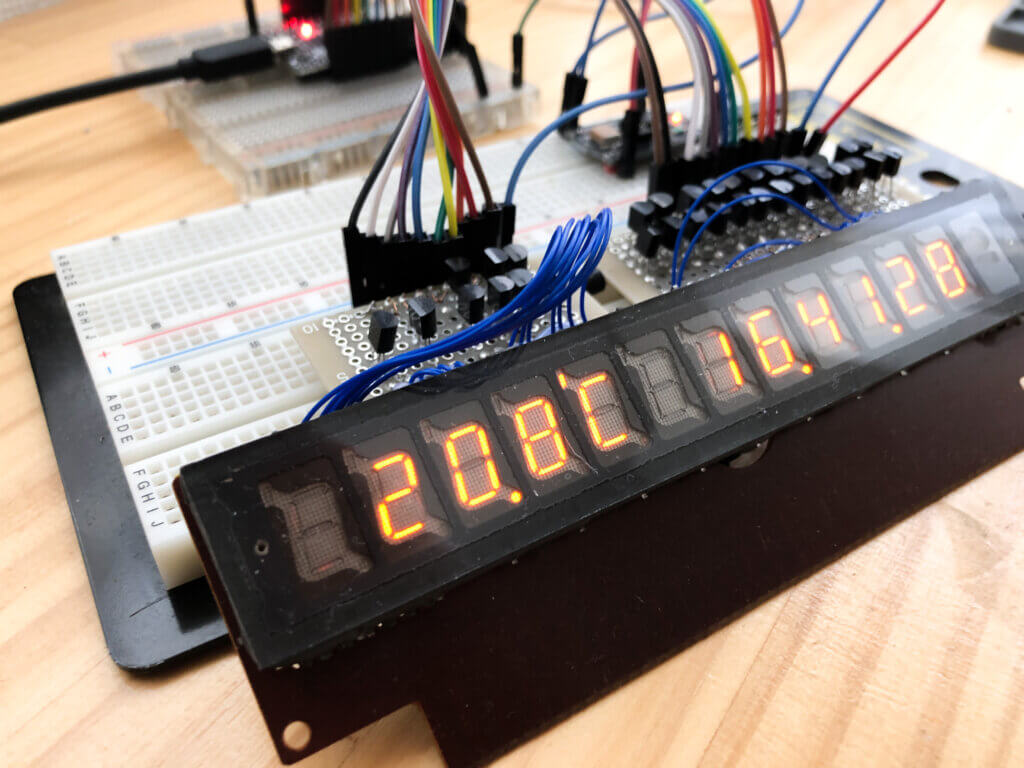I added a thermometer to an indicator that was manufactured during the short period before the transition from Nixie tubes to VFDs.

## SEPTANIX J4923A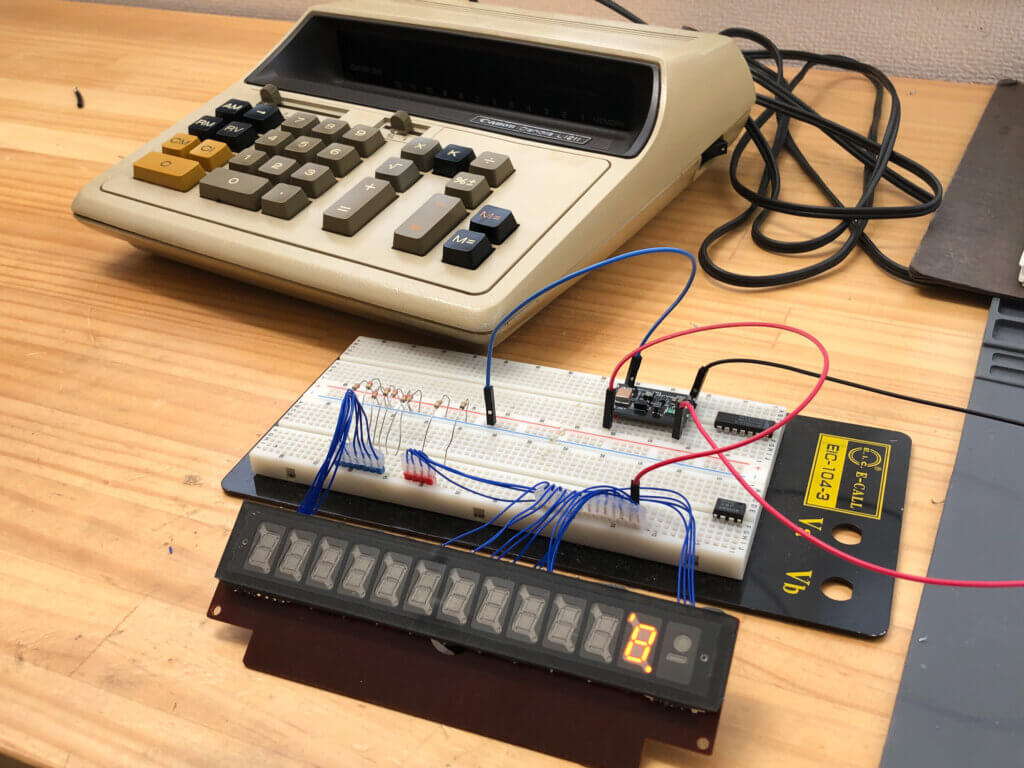This orange indicator was used in the display of an old 1970's desktop calculator called the Canon Canola L1211. It was introduced as an improved version of the Nixie tube, and although it is a 7-segment type indicator, the lighting method is similar to that of the Nixie tube. For more information about them, please see here.

I made an NTP calendar & clock that controls this display unit with ESP32 to get the time from the Internet and display it.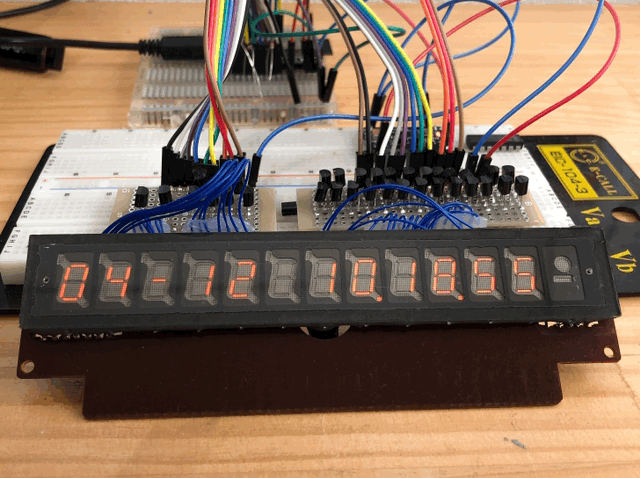This time, we would like to add a thermometer function to this NTP Calendar & Clock.

### Temperature sensor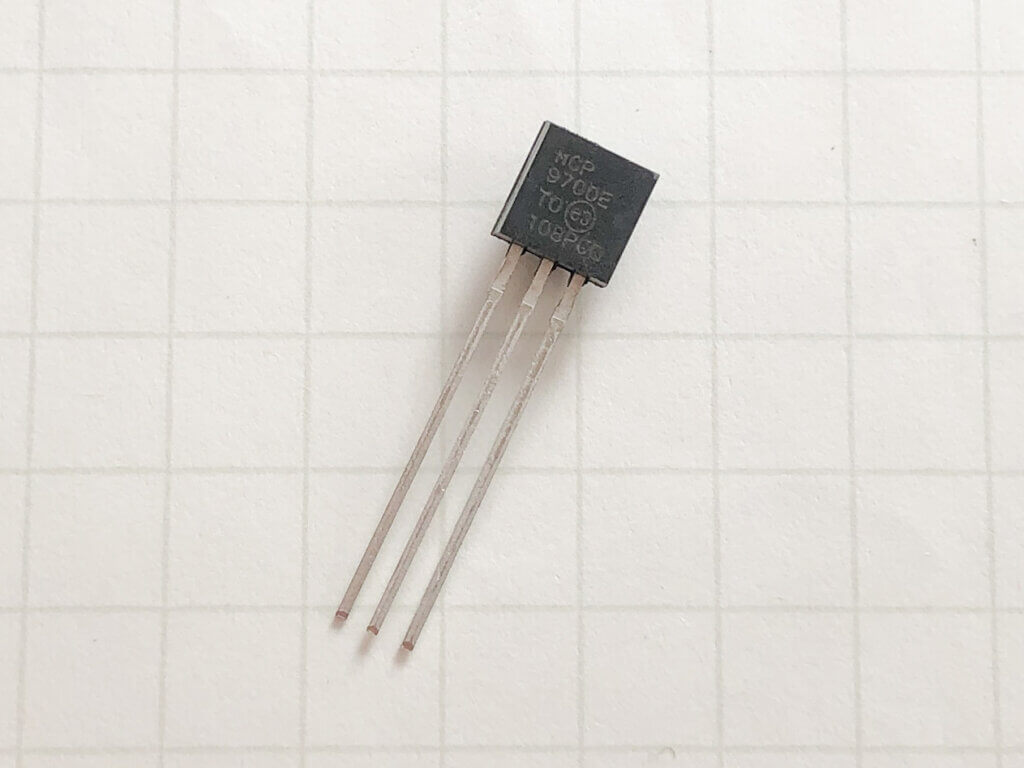Since all the digital IO of the ESP32 has been used up for controlling the display unit, we cannot add a digital communication type temperature sensor. Therefore, we would like to use an analog output type temperature sensor to measure the temperature by reading the voltage with the A/D converter of the ESP32.

For the analog temperature sensor, I used Microchip's MCP9700E/TO.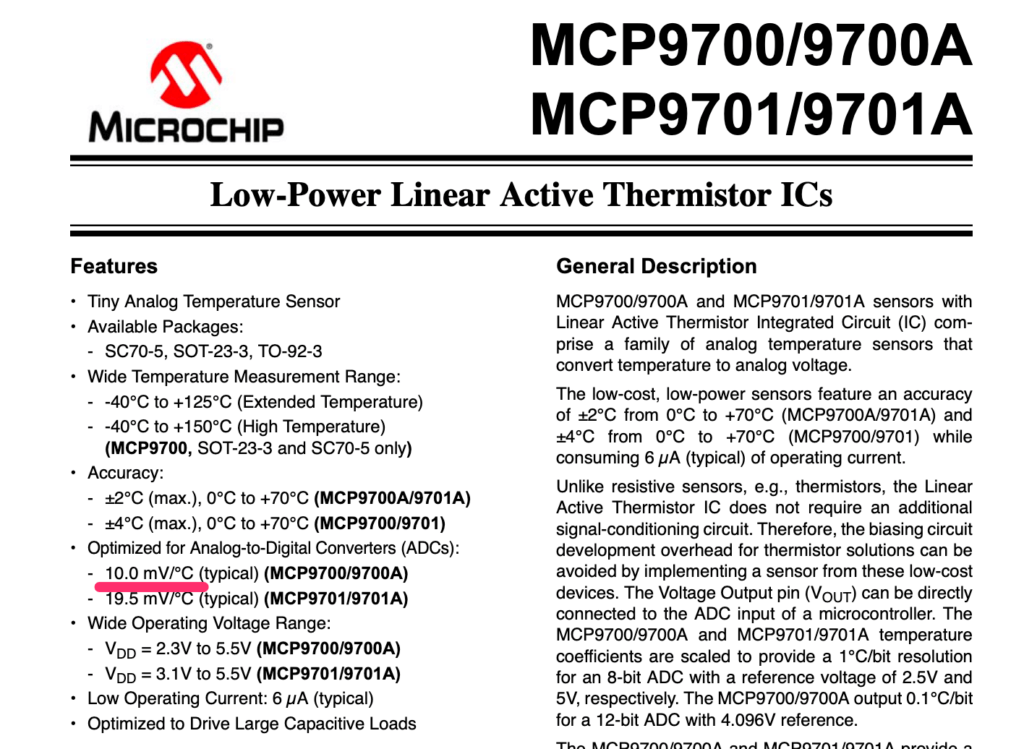This temperature sensor can measure from -40 to 125 degrees Celsius, and the output voltage increases by 10mV for every 1 degree increase in temperature. It is an easy to understand sensor.

### Converting from ESP32's A/D value to voltage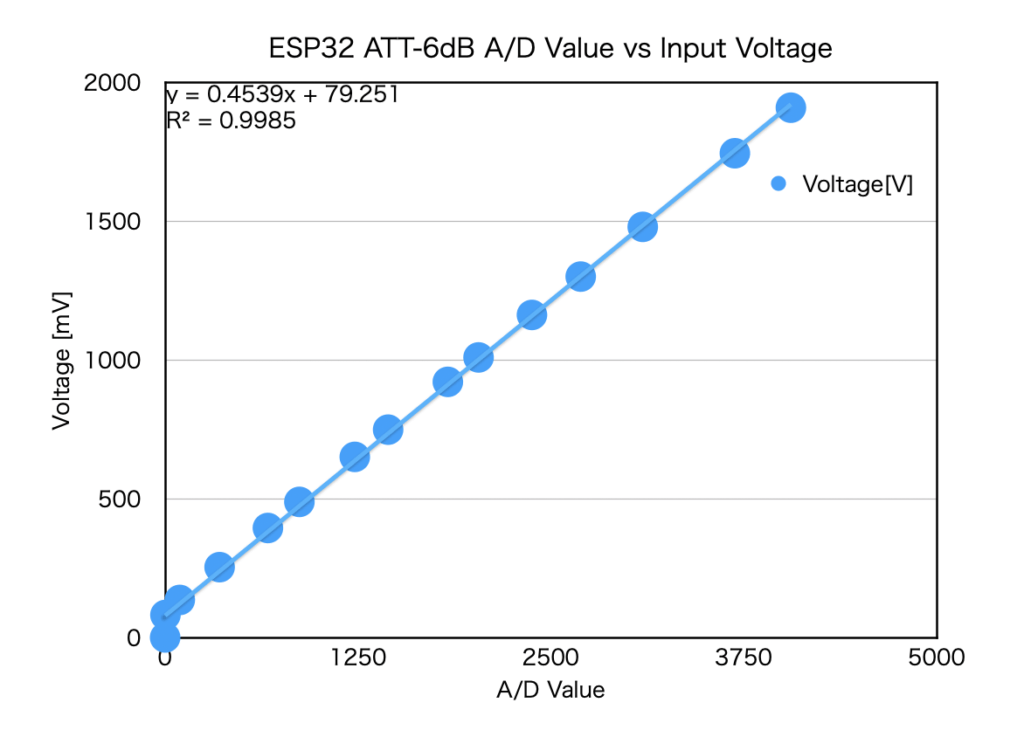Since we need to measure the voltage with the A/D converter of the ESP32, we need to know the relationship between the A/D value of the ESP32 and the input voltage.

The A/D converter of the ESP32 has a built-in attenuator function in the input stage, and the maximum voltage that can be measured depends on the attenuator setting.

In this case, since the maximum voltage output from the temperature sensor is about 2 V, we set the attenuator to -6 dB so that the maximum A/D measurement value would be about 2 V.

With this setting, I measured the A/D value by varying the input voltage of the A/D converter from 0V to 1.9V, and made a graph. The graph above is the result.

From this graph, a linear approximation showed that the voltage can be calculated from the A/D value using the following equation.

Voltage [mV] = 0.4539 × A/D value + 79.251

Using this formula, we can measure the voltage of the sensor.

### Conversion from voltage to temperature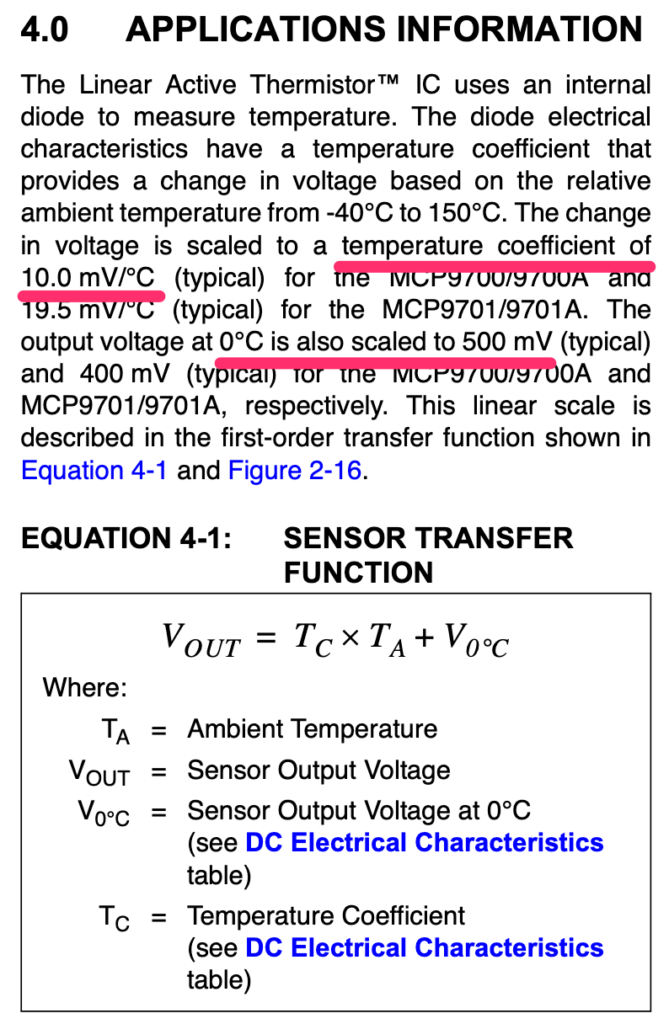From the datasheet, the output voltage of the MCP9700 is

Vout [mV] = 10 [mV] × temperature [°C] + 500 [mV]

Vout [mV] = 10 [mV] × temperature [°C] + 500 [mV]

Converting this equation to the temperature = equation, we get

Temperature [°C] = ( Vout [mV] - 500 [mV] ) / 10 [mV]

The result is as follows. By substituting the voltage value calculated from the A/D value into Vout in this equation, we can find the temperature.

## Circuit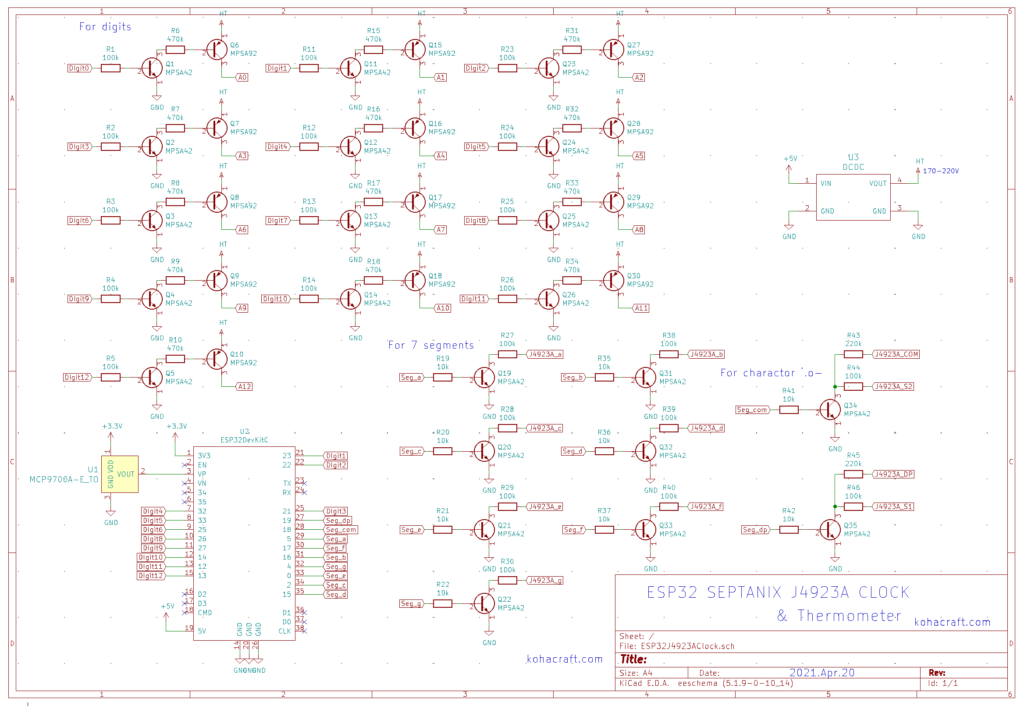I just added a temperature sensor MCP9700 to the circuit I made the other day. I connected the power supply of the sensor to 3.3V of the ESP32 and the output of the temperature sensor to pin 36 of the ESP32.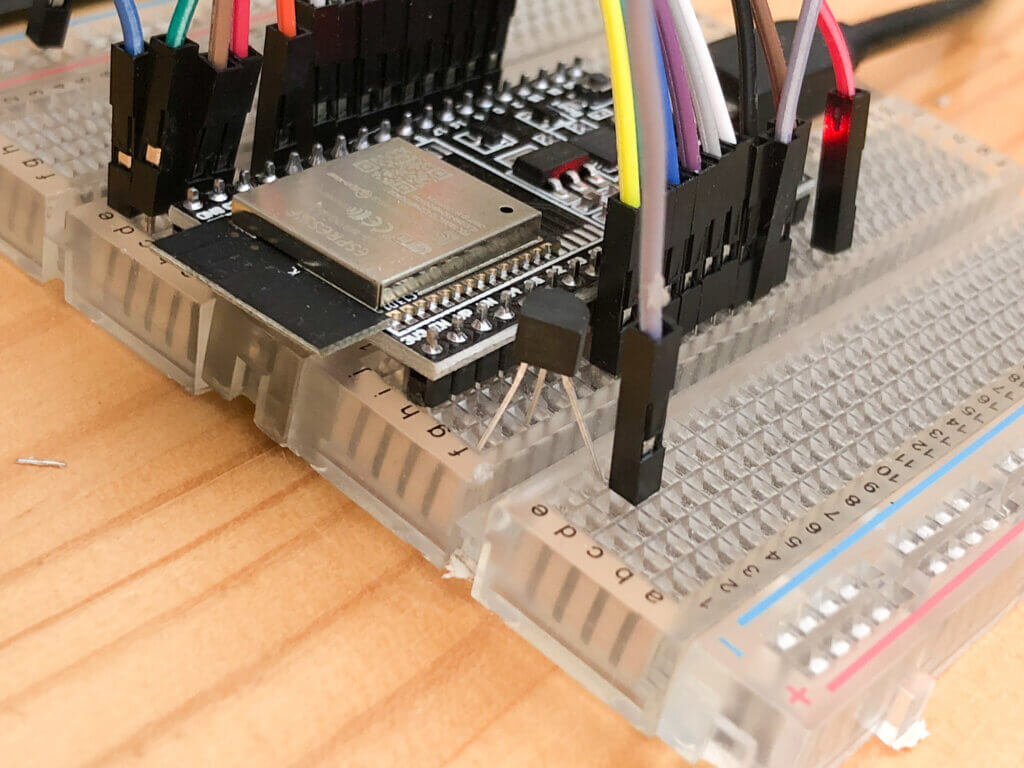The transistor-like component is the temperature sensor.

## Program

.
The code for the part that measures the temperature is as follows.

```  long d = 0;
for (int i = 0; i < 1000; i++)
{
}
double ad_value = (double)d / 1000.0;
temperature = ( ad_voltage - 500.0 ) / 10.0; // ['c]
```

The A/D value is measured 1000 times and the average value is used. The value is converted to a voltage using the formula obtained earlier, and further converted to a temperature.

The task is used to update the temperature once a second.

The entire program can be found here.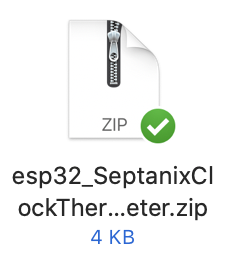## Thermometer complete!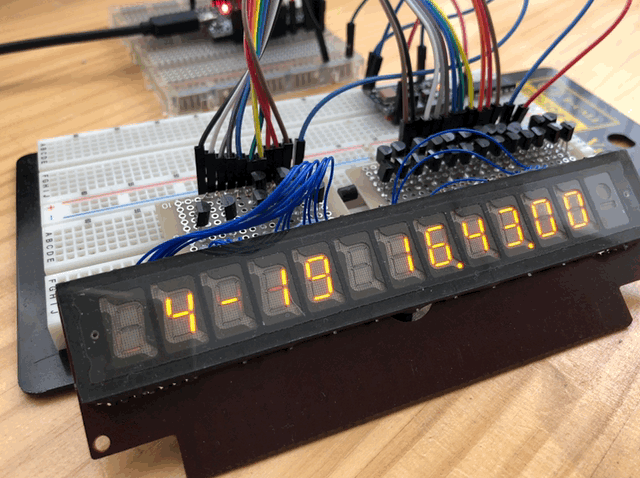I made it so that the calendar and thermometer switch every 5 seconds. The display of °C is represented by a combination of the letters ' and C.

Like the Nixie tube, this orange light has a certain charm that makes you keep staring at it.

The project using the display of a 1970's desktop calculator, Canon Canola L1211, is now complete. I now have a nice calendar, clock, and thermometer.

The Canon Canola series of desktop calculators with this indicator are sometimes sold on Yahoo! If you are interested, why don't you check it out? Some of the indicators are VDF instead of orange, so be careful when looking for one.